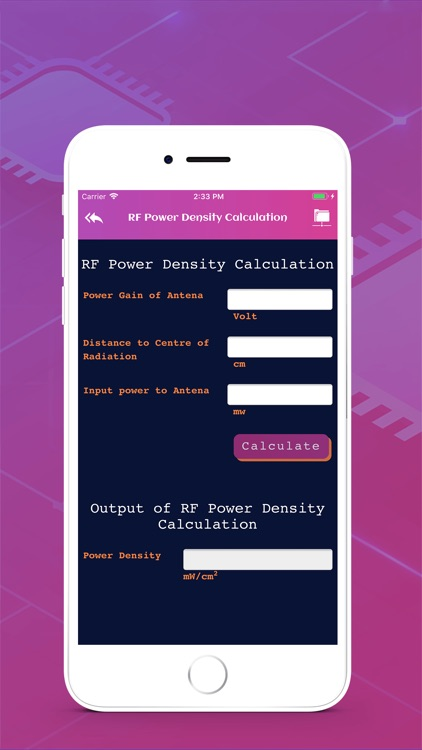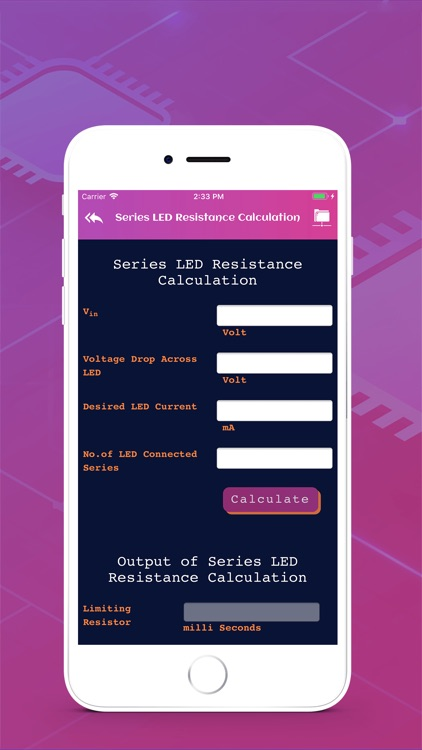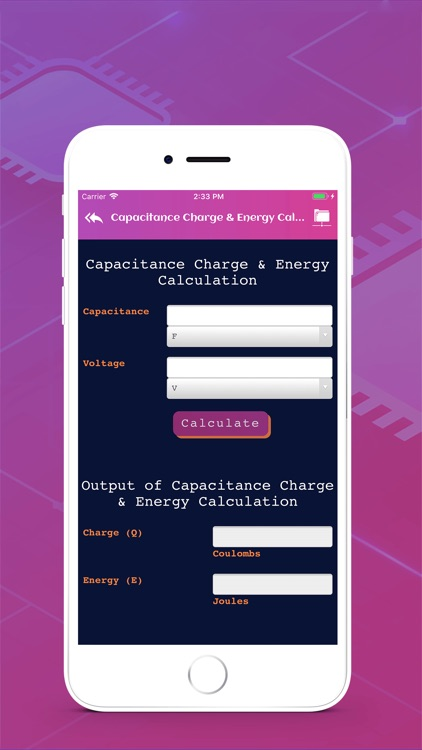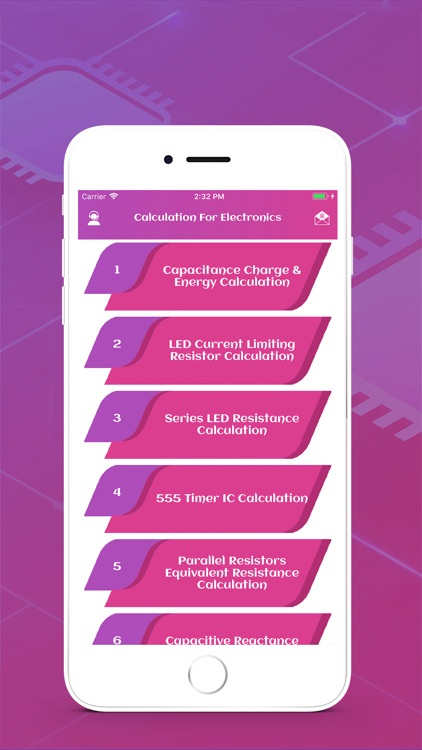## Calculation For Electronics app is a very useful app for electrical engineering students and electronics hobbyist# Calculation For Electronics

by John MoralezCalculation For Electronics app is a very useful app for electrical engineering students and electronics hobbyist.### App Details

Version
1.2
Rating
NA
Size
24Mb
Genre
Education Utilities
Last updated
July 18, 2019
Release date
July 5, 2019

### App Screenshots### App Store Description

Calculation For Electronics app is a very useful app for electrical engineering students and electronics hobbyist.

Calculation For Electronics is a utility application consisting of various calculators.

- Capacitance Charge & Energy Calculation
- LED Current Limiting Resistor Calculation
- Series LED Resistance Calculation
- 555 Timer IC Calculation
- Parallel Resistors Equivalent Resistance Calculation
- Capacitive Reactance Calculator
- RF Power Density Calculation
- RLC Circuit Frequency Calculation
- Potential Divider Output Voltage Calculation
- Microstrip Impedance Calculation
- Differential Microstrip Impedance Calculation
- Wire Length & Coil Frequency Calculation
- Zener Diode Power Rating Calculation
- Skin Effect Calculation
- 555 Timer IC Calculation
- OHM's Law Calculator
- Microstrip Impedance Calculation
- Bandwidth Calculation